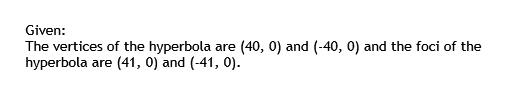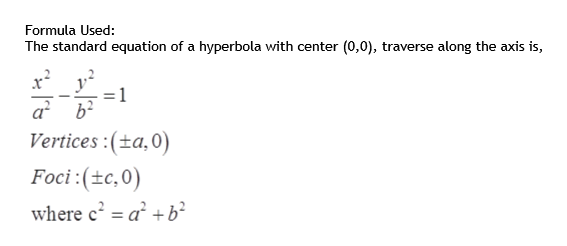# For Exercise, write the standard form of the equation of the hyperbola subject to the given conditions. Vertices: (40, 0), (−40, 0); Foci: (41, 0), (−41, 0)

Question
2 views

For Exercise, write the standard form of the equation of the hyperbola subject to the given conditions.

Vertices: (40, 0), (−40, 0); Foci: (41, 0), (−41, 0)

check_circle

Step 1

To determine the standard equation of the hyperbola.

Step 2

Given:

The vertices of the hyperbola are (40, 0) and (-40, 0) and the foci of the hyperbola are (41, 0) and (-41, 0).help_outlineImage TranscriptioncloseGiven: The vertices of the hyperbola are (40, 0) and (-40, 0) and the foci of the hyperbola are (41, 0) and (-41, 0). fullscreen
Step 3

Formula Used:

The standard equation of a hyperbola with cente...help_outlineImage TranscriptioncloseFormula Used: The standard equation of a hyperbola with center (0,0), traverse along the axis is, a b? Vertices :(±a,0) Foci:(±c,0) where c = a² + b² %3D fullscreen

### Want to see the full answer?

See Solution

#### Want to see this answer and more?

Solutions are written by subject experts who are available 24/7. Questions are typically answered within 1 hour.*

See Solution
*Response times may vary by subject and question.
Tagged in

### Algebra